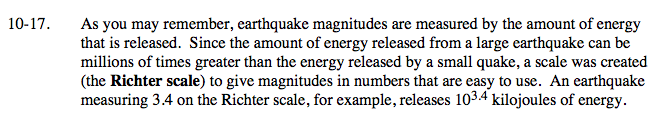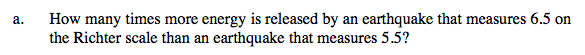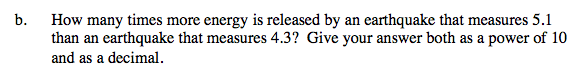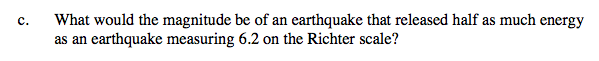### Home > A2C > Chapter 10 > Lesson 10.1.1 > Problem10-17

10-17.
1. As you may remember, earthquake magnitudes are measured by the amount of energy that is released. Since the amount of energy released from a large earthquake can be millions of times greater than the energy released by a small quake, a scale was created (the Richter scale) to give magnitudes in numbers that are easy to use. An earthquake measuring 3.4 on the Richter scale, for example, releases 103.4 kilojoules of energy. Homework Help ✎

1. How many times more energy is released by an earthquake that measures 6.5 on the Richter scale than an earthquake that measures 5.5?

2. How many times more energy is released by an earthquake that measures 5.1 than an earthquake that measures 4.3? Give your answer both as a power of 10 and as a decimal.

3. What would the magnitude be of an earthquake that released half as much energy as an earthquake measuring 6.2 on the Richter scale?Solve the equation:
x · 105.5 = 106.5

10 times more energy.100.8 = 6.3105.9